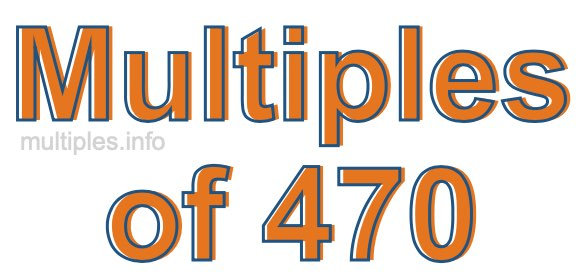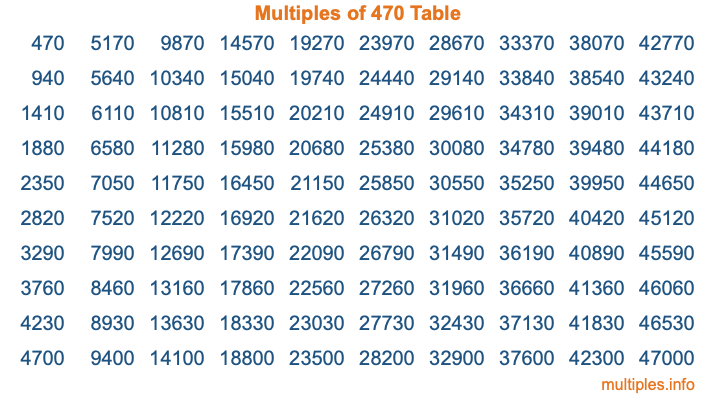Multiples of 470Welcome to the Multiples of 470 page. Here we will first teach you everything you will ever need to know about the multiples of 470, and then give you a study guide summary of everything we taught you to make sure you remember it all. Use this page to look up facts and learn information about the multiples of 470. This page will make you a multiples of four hundred seventy expert!

Definition of Multiples of 470
Multiples of 470 are all the numbers that when divided by 470 equal an integer. Each of the multiples of 470 are called a multiple. A multiple of 470 is created by multiplying 470 by an integer.

Therefore, to create a list of multiples of 470, you start with 1 multiplied by 470, then 2 multiplied by 470, then 3 multiplied by 470, and so on for as long as you want. Thus, the list of the first five multiples of 470 is 470, 940, 1410, 1880, and 2350. To see a larger list of multiples of 470, see the printable image of Multiples of 470 further down on this page. We also have a category where you can choose any nth multiple of 470.

Multiples of 470 Checker
The Multiples of 470 Checker below checks to see if any number of your choice is a multiple of 470. In other words, it checks to see if there is any number (integer) that when multiplied by 470 will equal your number. To do that, we divide your number by 470. If the the quotient is an integer, then your number is a multiple of 470.

Is  a multiple of 470?

Least Common Multiple of 470 and ...
A Least Common Multiple (LCM) is the lowest multiple that two or more numbers have in common. This is also called the smallest common multiple or lowest common multiple and is useful to know when you are adding our subtracting fractions. Enter one or more numbers below (470 is already entered) to find the LCM.

Check out our LCM Calculator if you need more details about the Least Common Multiple or if you need the LCM for different numbers for adding and subtraction fractions.

nth Multiple of 470
As we stated above, 470 is the first multiple of 470, 940 is the second multiple of 470, 1410 is the third multiple of 470, and so on. Enter a number below to find the nth multiple of 470.

th multiple of 470

Multiples of 470 vs Factors of 470
470 is a multiple of 470 and a factor of 470, but that is where the similarities end. All postive multiples of 470 are 470 or greater than 470. All positive factors of 470 are 470 or less than 470.

Below is the beginning list of multiples of 470 and the factors of 470 so you can compare:

Multiples of 470: 470, 940, 1410, 1880, 2350, etc.

Factors of 470: 1, 2, 5, 10, 47, 94, 235, 470

As you can see, the multiples of 470 are all the numbers that you can divide by 470 to get a whole number. The factors of 470, on the other hand, are all the whole numbers that you can multiply by another whole number to get 470.

It's also interesting to note that if a number (x) is a factor of 470, then 470 will also be a multiple of that number (x).

Multiples of 470 vs Divisors of 470
The divisors of 470 are all the integers that 470 can be divided by evenly. Below is a list of the divisors of 470.

Divisors of 470: 1, 2, 5, 10, 47, 94, 235, 470

The interesting thing to note here is that if you take any multiple of 470 and divide it by a divisor of 470, you will see that the quotient is an integer.

Multiples of 470 Table
Below is an image of the first 100 multiples of 470 in a table. The table is in chronological order, column by column. The first column has the first ten multiples of 470, the second column has the next ten multiples of 470, and so on.The Multiples of 470 Table is also referred to as the 470 Times Table or Times Table of 470. You are welcome to print out our table for your studies.

Negative Multiples of 470
Although not often discussed or needed in math, it is worth mentioning that you can make a list of negative multiples of 470 by multiplying 470 by -1, then by -2, then by -3, and so on, to get the following list of negative multiples of 470:

-470, -940, -1410, -1880, -2350, etc.

Multiples of 470 Summary
Below is a summary of important Multiples of 470 facts that we have discussed on this page. To retain the knowledge on this page, we recommend that you read through the summary and explain to yourself or a study partner why they hold true.

There are an infinite number of multiples of 470.

A multiple of 470 divided by 470 will equal a whole number.

470 divided by a factor of 470 equals a divisor of 470.

The nth multiple of 470 is n times 470.

The largest factor of 470 is equal to the first positive multiple of 470.

470 is a multiple of every factor of 470.

470 is a multiple of 470.

A multiple of 470 divided by a divisor of 470 equals an integer.

470 divided by a divisor of 470 equals a factor of 470.

Any integer times 470 will equal a multiple of 470.

Multiples of a Number
Here you can get the multiples of another number, all with the same attention to detail as we did for multiples of 470 on this page.

Multiples of
Multiples of 471
Did you find our page about multiples of four hundred seventy educational? Do you want more knowledge? Check out the multiples of the next number on our list!